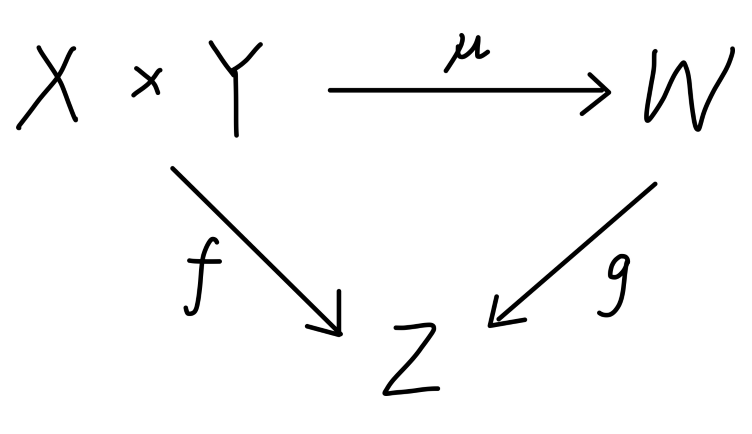## 张量

### 多重线性映射

$X, Y$$Z$ 为域 $\mathbb{K}$ 上的三个线性空间。若映射 $f : X ×Y \rightarrow Z$ 满足：

\begin{aligned} & \forall x_1,x_2,x \in X,\ \forall y_1,y_2,y \in Y,\ \forall \lambda_1,\lambda_2 \in \mathbb{K}\\ &f(\lambda_1x_1 + \lambda_2x_2,y) = \lambda_1f(x_1,y) + \lambda_2f(x_2,y)\\ &f(x,\lambda_1y_1 + \lambda_2y_2) = \lambda_1f(x,y_1) + \lambda_2f(x,y_2)\\ \end{aligned}

$f : X_1\times X_2 \times\cdots \times X_r \rightarrow Z$

$\mathscr{L}^{(r)} (X_1,··· ,X_r;Z)$

\begin{aligned} &(f + g)(x_1,··· ,x_r) = f(x_1,··· ,x_r) + g(x_1,··· ,x_r)\\ &(\lambda f)(x_1,··· ,x_r) = \lambda f(x_1,··· ,x_r)\\ \end{aligned}

### 张量积

#### 线性空间的张量积

$X, Y , W$ 是数域 $\mathbb{K}$ 上的三个线性空间。$\mu : X × Y \rightarrow W$ 是一个双线性映射。若对任意的 $\mathbb{ K}$ 上的线性空间 $Z$ 和任意的双线性映射 $f$，都存在唯一的线性映射 $g : W \rightarrow Z$ 使得：

$f = g \circ \mu$$X, Y$ 是数域 $\mathbb{K}$ 上的两个线性空间。则它们的张量积存在，而且在同构的意义下是唯一的。

$x^* \in X ^*, y^*\in Y^*$ 分别是 $X$$Y$ 上的线性函数，我们可以定义它们的 张量积，记为 $x^* \otimes y^*$，其定义如下：

$(x ^* \otimes y^* )(x',y' ) = ⟨x' ,x ^* ⟩⟨y',y^* ⟩=x^* (x') y^*(y'),\quad \forall x' \in X,\ \forall y' \in Y$

$⟨x' ,x ^* ⟩ = x'(x^*) = x^*(x')$

$x ^* \otimes y^ * \in \mathscr{L}^{(2)} (X,Y ;\mathbb{K})$

$C_{i\alpha } e^{*i} \otimes h^ {*\alpha }$

$\dim(X^* \otimes Y^* ) = nm$

$X,Y$ 是两个线性空间，则：

$X^* \otimes Y^* = \mathscr{L}^{(2)}(X,Y;\mathbb{K})$

$X^* \otimes Y^*$ 中的元素均为双重线性映射可知。

$X \otimes Y = \mathscr{L}^{(2)}(X^*,Y^*;\mathbb{K})$

\begin{aligned} \mu : &X \times Y \rightarrow \mathscr{L}^{(2)} (X^* ,Y^ * ;\mathbb{K})\\ & (x,y) \mapsto x \otimes y\\ \end{aligned}

#### 多重线性函数的张量积

$x$$y$ 分别是 $r$-重线性函数和 $s$-重线性函数，即：

\begin{aligned} &x\in\mathscr{L}^{(r)}(X_ 1 ,··· ,X_r ;\mathbb{K})\\ &y\in\mathscr{L}^{(s)}(Y_1 ,··· ,Y_s ;\mathbb{K})\\ \end{aligned}

\begin{aligned} &x\otimes y(u_1 ,··· ,u_r ;v_1 ,··· ,v_s ) = x(u_1 ,··· ,u_r ) y(v_1 ,··· ,v_s )\\ &\quad \forall u_i \in X_i ,\ i = 1,···,r \quad \forall v_j \in Y_j ,\ j = 1,···,s\\ \end{aligned}

$x \otimes y : X_1 × ··· × X_r × Y_1 × ···Y_s \rightarrow \mathbb{K}$

$(x \otimes y) \otimes z = x \otimes (y \otimes z)$

$x\otimes y\otimes z$

### 张量

$X_s^r=\underbrace{X \otimes ··· \otimes X}_{r}\otimes\underbrace{X^* \otimes ··· \otimes X^* } _ {s}$

$r$$X$$s$$X^*$ 作张量积，其中 $X^*$$X$ 的对偶空间。

$X\otimes X \otimes X^*\quad X\otimes X^* \otimes X\quad X^*\otimes X \otimes X$

\begin{aligned} &X_0^0=\mathbb{K}\\ &X_0^1=X\\ &X^0_1=X^* \\ &X_0^r=\underbrace{X\otimes\cdots\otimes X}_{r}\\ &X_s^0=\underbrace{X^* \otimes\cdots\otimes X^* }_{s}\\ \end{aligned}

$\dim (X_{s}^{r}) = (\dim(X))^{r+s}$

\begin{aligned} X_s^r&=\mathscr{L}^{(r+s)}(\underbrace{X^*,\cdots,X^* }_{r},\underbrace{X,\cdots, X}_{s};\mathbb{K})\\ &=\mathscr{L}^{(1)}(\underbrace{X^* \otimes\cdots\otimes X^* }_{r}\otimes\underbrace{X\otimes\cdots\otimes X}_{s};\mathbb{K})\\ \end{aligned}

• $X\times X^*\rightarrow\mathbb{K}$ 的双线性函数 $\mathscr{L}^{(2)}(\cdot,\cdot;\mathbb{K})$
• $X\rightarrow X^*$ 的线性函数 $\mathscr{L}^{(2)}(\cdot,X^*;\mathbb{K})$
• $X^* \rightarrow X$ 的线性函数 $\mathscr{L}^{(2)}(X,\cdot;\mathbb{K})$

#### 张量在基底下的表示

$x \in X^r_s$，则我们可以将其表示为：

$x = x^{i_1 ···i_r}_{\qquad j_1 ···j_s} e_{i_1} \otimes\cdots \otimes e_{i_r} \otimes e^{*j_1} \otimes \cdots \otimes e^{*j_s}$

$x^{i_1 ···i_r}_{\qquad j_1 ···j_s} \in\mathbb{K}$

$\{e_{i_1} \otimes \cdots\otimes e_{i_r} \otimes e^{*j_1} \otimes \cdots\otimes e^{*j_s}\}$

$e_i \rightarrow \tilde{e}_i = A^{\ \ k}_ie_k$

$e^{* i} \rightarrow \tilde{e}^{* i} = (A^{-1})^{\ \ i}_ke^{* k}$

$x^{i_1 ···i_r}_{\qquad j_1 ···j_s}=\tilde{x}^{k_1 ···k_r}_{\qquad l_1 ···l_s}A_{k_1}^{\ \ i_1}\cdots A_{k_r}^{\ \ i_r}(A^{-1}) _ {j_1}^{\ \ l_1}\cdots (A^{-1}) _ {j_r}^{\ \ l_r}$

#### 张量的缩并

$C : X^r_s\rightarrow X^{r-1}_{s-1}$

$1\leqslant l\leqslant s, 1\leqslant m \leqslant s$，张量 $x\in X^r_s$，那么 $C(x)\in X^{r-1}_{s-1}$，对应的缩并运算定义为：

\begin{aligned} &C_l^{\ \ m} (x)(f^1 ,··· ,f^{r-1} ,u_1 ,··· ,u_{s-1} )\\=& \delta_i^{\ \ j} x(f ^1 ,··· ,f^{m-1} ,e^{*i} ,f^m ···f^{r-1},u_1 ,··· ,u_{l-1},e_j ,u_l ,···u_{s-1}) \end{aligned}

$f^i \in X^* ,i = 1,··· ,r - 1; u_j \in X ,j = 1,··· ,s - 1$

\begin{aligned} &\delta_i^{\ \ j} x(f ^1 ,··· ,f^{m-1} ,e^{*i} ,f^m ···f^{r-1},u_1 ,··· ,u_{l-1},e_j ,u_l ,···u_{s-1}) \\ =& x^{i_1\cdots i_{m-1}ki_{m}\cdots i_{r-1}}_{\qquad\qquad\quad\ \ j_1\cdots j_{l-1}tj_l\cdots j_{s-1}} \delta_i^{\ \ j} [ e_{i_1}\otimes \cdots \otimes e_{i_{m-1}}\otimes e_{k} \otimes e_{m} \otimes \cdots \otimes e_{i_{r-1}}\\ &\otimes e^{*j_1}\otimes \cdots \otimes e^{*j_{l-1}}\otimes e^{*t}\otimes e^{*j_{l}}\otimes \cdots \otimes e^{*j_{s-1}}\\ &(f ^1 ,··· ,f^{m-1} ,e^{*i} ,f^m ···f^{r-1},u_1 ,··· ,u_{l-1},e_j ,u_l ,···u_{s-1})]\\ =&x^{i_1\cdots i_{m-1}ki_{m}\cdots i_{r-1}}_{\qquad\qquad\quad\ \ j_1\cdots j_{l-1}tj_l\cdots j_{s-1}} [ e_{i_1}\otimes\cdots \otimes e_{i_{r-1}}\otimes e^{*j_1}\otimes \cdots \otimes e^{*j_{s-1}}\\ &(f^1,\cdots,f^{r-1},u_1,\cdots,u_{s-1})]\delta_i^{\ \ j}\langle e_k,e^{*i}\rangle\langle e_j,e^{*t}\rangle\\ =&x^{i_1\cdots i_{m-1}ii_{m}\cdots i_{r-1}}_{\qquad\qquad\quad\ \ j_1\cdots j_{l-1}jj_l\cdots j_{s-1}} \delta_{i}^{\ \ j} [ e_{i_1}\otimes\cdots \otimes e_{i_{r-1}}\otimes e^{*j_1}\otimes \cdots \otimes e^{*j_{s-1}}\\ &(f^1,\cdots,f^{r-1},u_1,\cdots,u_{s-1})]\\ \end{aligned}

$(C^m_l(x))^{i_1\cdots i_{r-1}}_{\qquad\ \ \ j_1\cdots j_{s-1}} = \delta_{i}^{\ \ j}x^{i_1\cdots i_{m-1}ii_{m}\cdots i_{r-1}}_{\qquad\qquad\quad\ \ j_1\cdots j_{l-1}jj_l\cdots j_{s-1}}$

$C_{1}^{\ \ 1}(x) = x^{i}_{\ \ i}$

#### 抽象指标

$x^{a_1 \cdots a_r}_{\qquad b_1 \cdots b_s}$

$x : X ^* × X ^* × X × X × X ^* \rightarrow \mathbb{K}$

• 等式左右出现的指标相同
• 相同指标的类型相同（上下位置相同）

• 张量积的抽象指标
两个张量的张量积 $x \otimes y$ 可表示为

$x^{a_1 \cdots a_r}_{\qquad b_1 \cdots b_s}\otimes y^{a_{r+1} \cdots a_{r+p}}_{\qquad\quad\ b_{s+1} \cdots b_{s+q}}$

$x^{a_1 \cdots a_r}_{\qquad b_1 \cdots b_s} y^{a_{r+1} \cdots a_{r+p}}_{\qquad\quad\ b_{s+1} \cdots b_{s+q}}$

• 基底的抽象指标形式
$\{e_i \}$$X$ 的一组基，$\{e^{*i} \}$ 为对偶基。则 $e_i$ 是逆变矢量，$e^{*i}$ 是协变矢量。用抽象指标形式表示为：

$(e_i)^a , (e^{*i})_ a$

• 缩并操作
重复的上下指标表示对这两个指标求缩并：

\begin{aligned} &(C^m_l(x) ) ^{a_1 \cdots a_{m-1} a_{m} \cdots a_{r-1}} _ {\qquad \qquad\qquad b_1 \cdots b_{l-1} b_l \cdots b_{ s-1}}\\ &\quad =x^{a_1 \cdots a_{m-1} aa_{m} \cdots a_{r-1}} _ {\qquad \qquad\qquad\ b_1 \cdots b_{l-1} ab_l \cdots b_{ s-1}} \end{aligned}

$\delta = \delta^{i}_{\ \ j}e_i \otimes e^{*j}$

$\delta^a_{\ \ b} = \delta^{i}_{\ \ j}(e_i)^a(e^{*j})_b$

$\delta^a_{\ \ b}u^b = u^a$